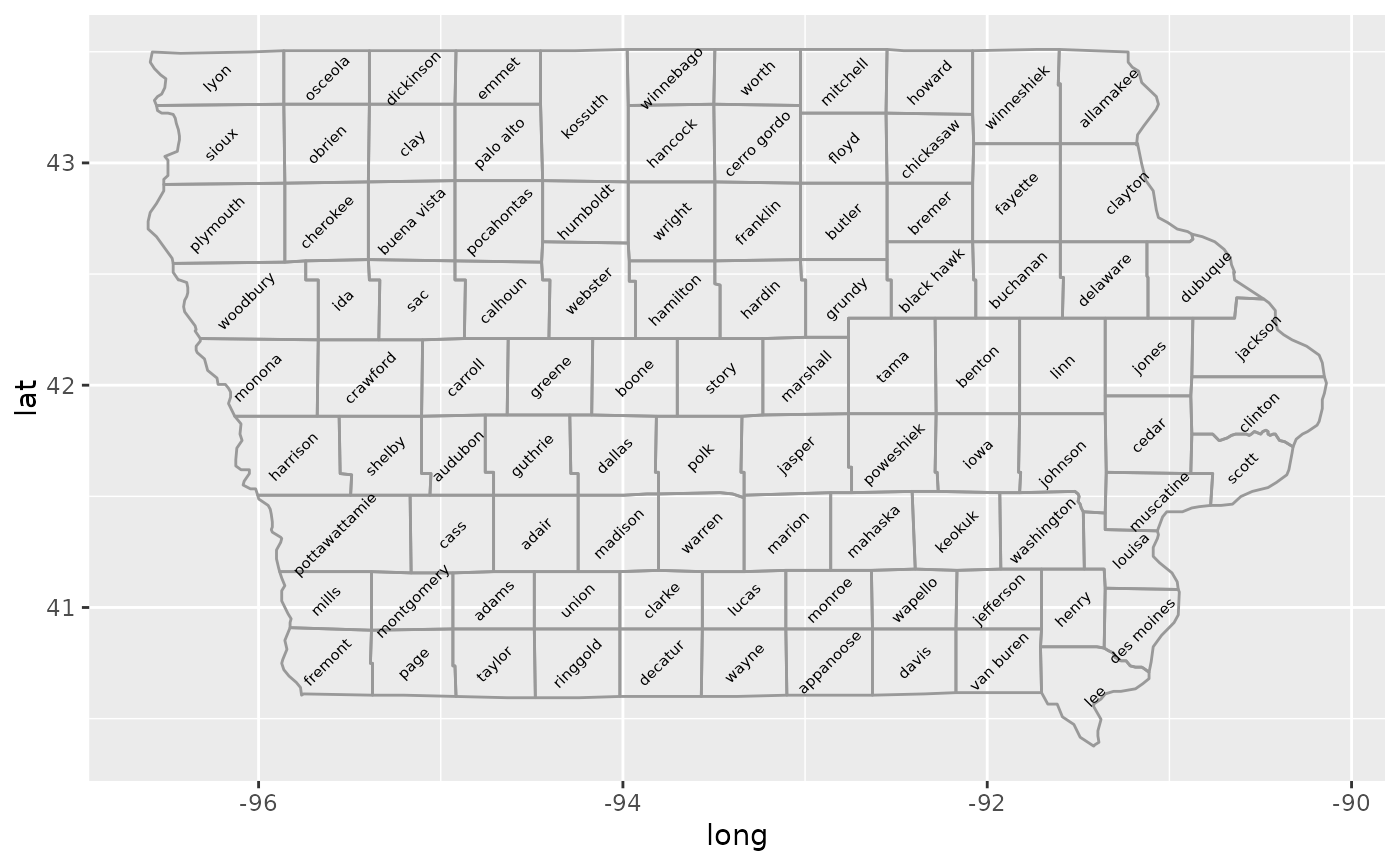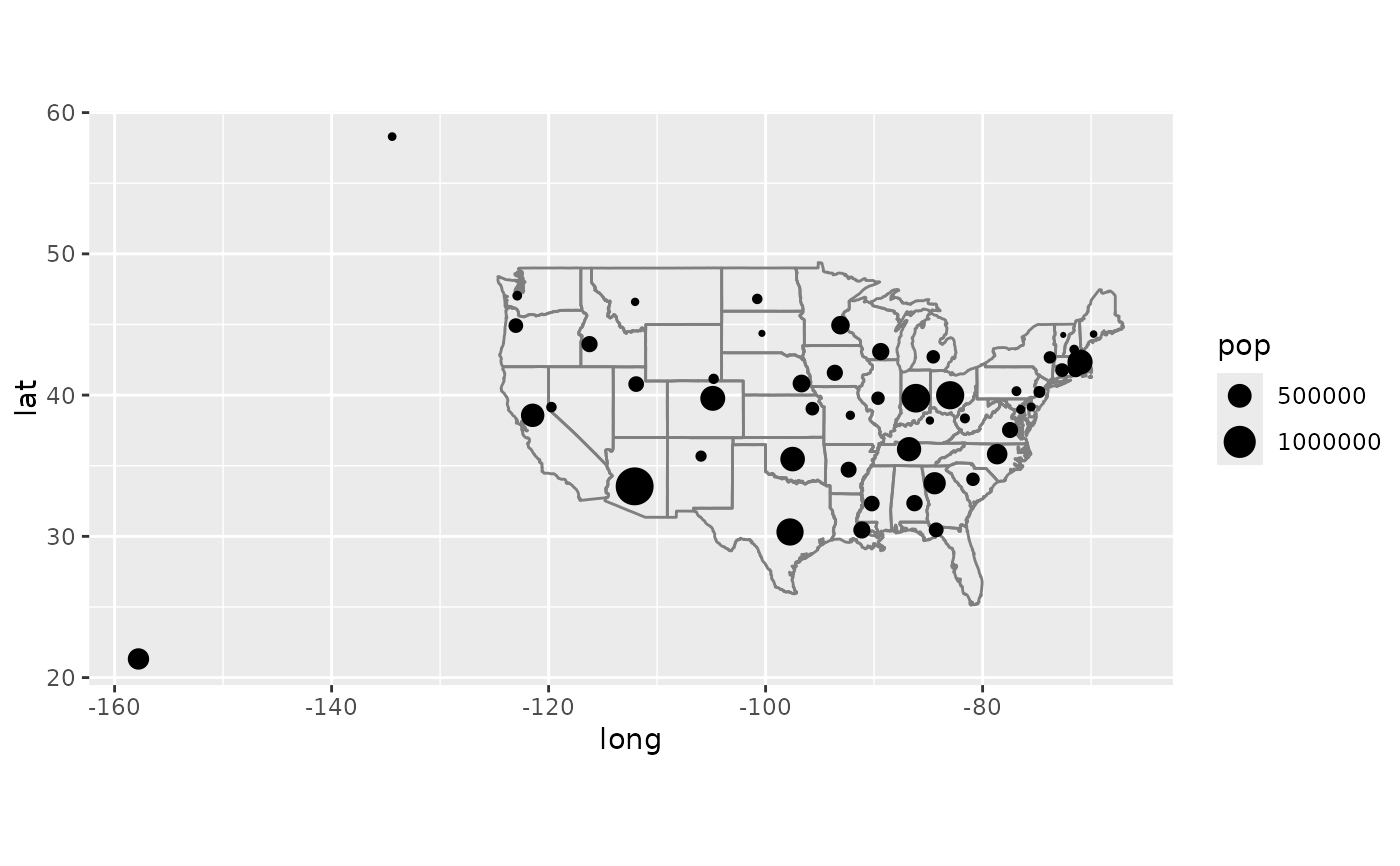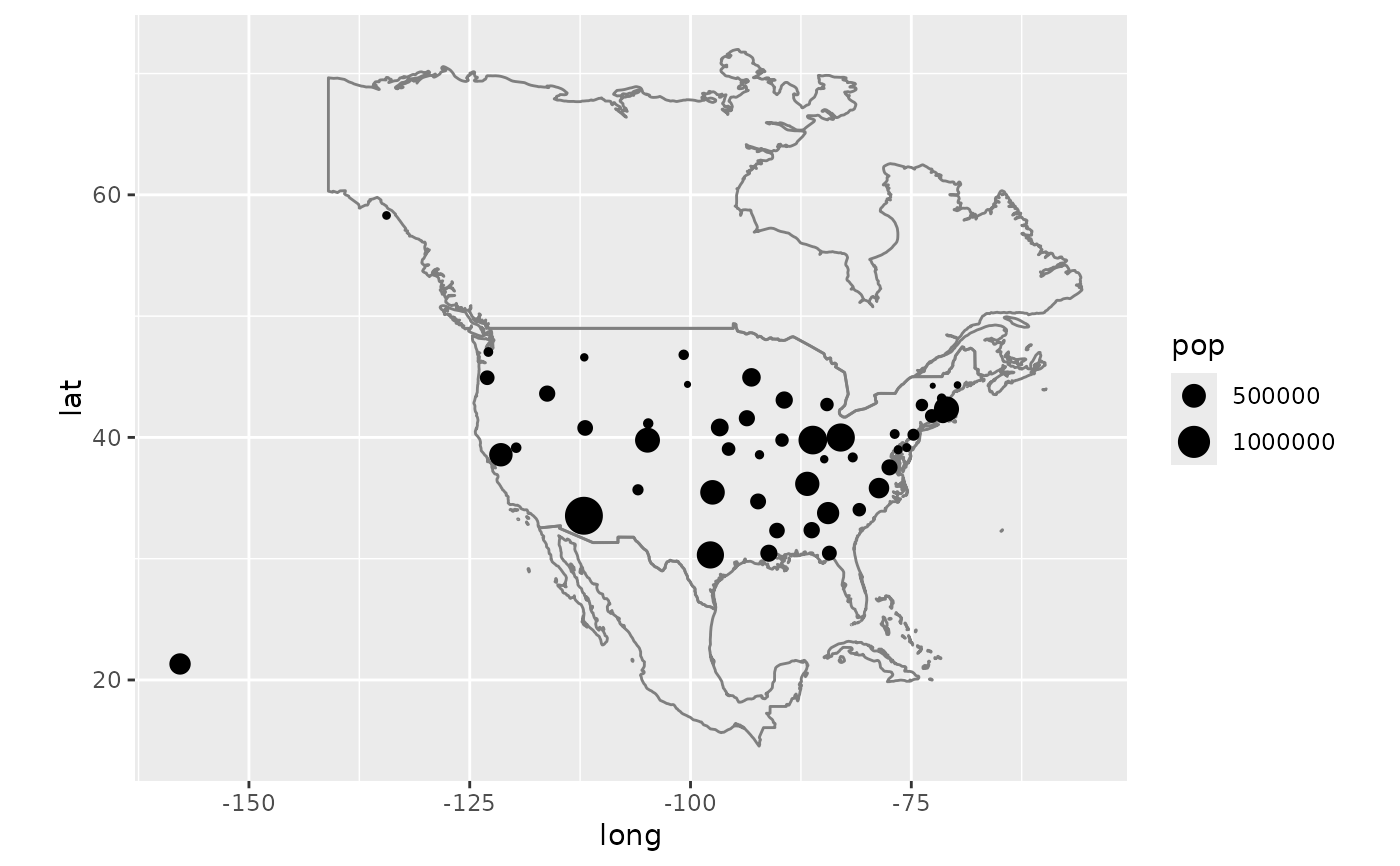This is a quick and dirty way to get map data (from the maps package) on to your plot. This is a good place to start if you need some crude reference lines, but you'll typically want something more sophisticated for communication graphics.

## Usage

borders(
database = "world",
regions = ".",
fill = NA,
colour = "grey50",
xlim = NULL,
ylim = NULL,
...
)

## Arguments

database

map data, see maps::map() for details

regions

map region

fill

fill colour

colour

border colour

xlim, ylim

latitudinal and longitudinal ranges for extracting map polygons, see maps::map() for details.

...

Arguments passed on to geom_polygon

rule

Either "evenodd" or "winding". If polygons with holes are being drawn (using the subgroup aesthetic) this argument defines how the hole coordinates are interpreted. See the examples in grid::pathGrob() for an explanation.

mapping

Set of aesthetic mappings created by aes() or aes_(). If specified and inherit.aes = TRUE (the default), it is combined with the default mapping at the top level of the plot. You must supply mapping if there is no plot mapping.

data

The data to be displayed in this layer. There are three options:

If NULL, the default, the data is inherited from the plot data as specified in the call to ggplot().

A data.frame, or other object, will override the plot data. All objects will be fortified to produce a data frame. See fortify() for which variables will be created.

A function will be called with a single argument, the plot data. The return value must be a data.frame, and will be used as the layer data. A function can be created from a formula (e.g. ~ head(.x, 10)).

stat

The statistical transformation to use on the data for this layer, as a string.

position

Position adjustment, either as a string, or the result of a call to a position adjustment function.

show.legend

logical. Should this layer be included in the legends? NA, the default, includes if any aesthetics are mapped. FALSE never includes, and TRUE always includes. It can also be a named logical vector to finely select the aesthetics to display.

inherit.aes

If FALSE, overrides the default aesthetics, rather than combining with them. This is most useful for helper functions that define both data and aesthetics and shouldn't inherit behaviour from the default plot specification, e.g. borders().

na.rm

If FALSE, the default, missing values are removed with a warning. If TRUE, missing values are silently removed.

## Examples

if (require("maps")) {

ia <- map_data("county", "iowa")
mid_range <- function(x) mean(range(x))
seats <- do.call(rbind, lapply(split(ia, ia$subregion), function(d) { data.frame(lat = mid_range(d$lat), long = mid_range(d$long), subregion = unique(d$subregion))
}))

ggplot(ia, aes(long, lat)) +
geom_polygon(aes(group = group), fill = NA, colour = "grey60") +
geom_text(aes(label = subregion), data = seats, size = 2, angle = 45)
}if (require("maps")) {
data(us.cities)
capitals <- subset(us.cities, capital == 2)
ggplot(capitals, aes(long, lat)) +
borders("state") +
geom_point(aes(size = pop)) +
scale_size_area() +
coord_quickmap()
}if (require("maps")) {
# Same map, with some world context
ggplot(capitals, aes(long, lat)) +
borders("world", xlim = c(-130, -60), ylim = c(20, 50)) +
geom_point(aes(size = pop)) +
scale_size_area() +
coord_quickmap()
}